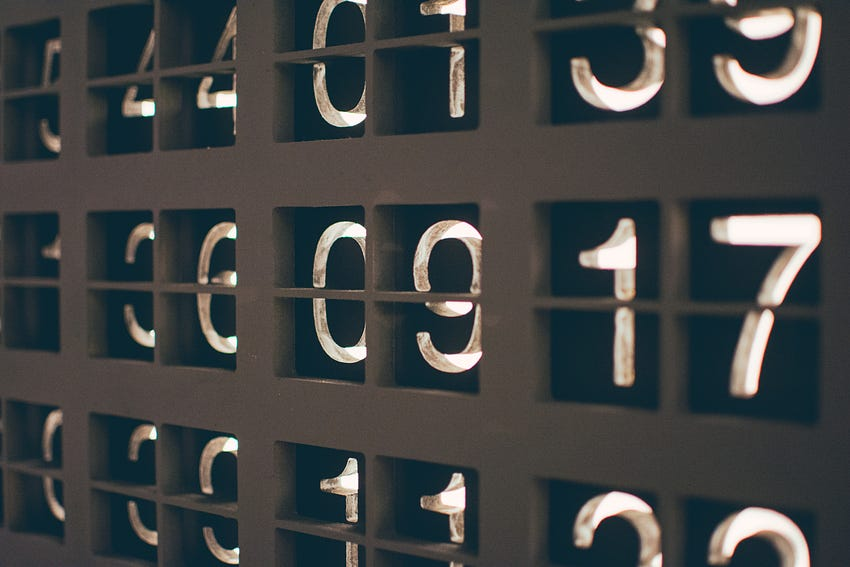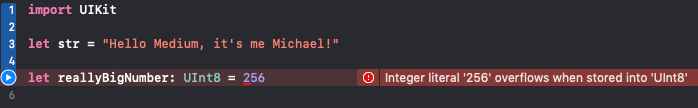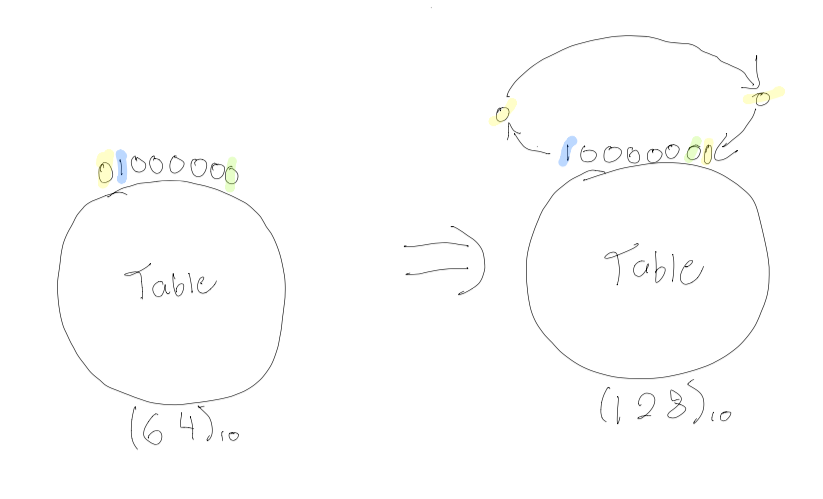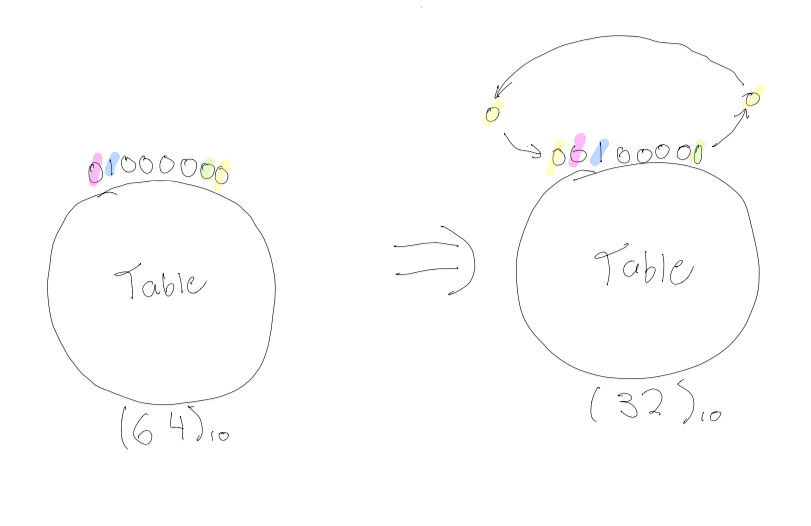# What is Bitwise Shifting?soconYou might have seen the advanced operators “<<” and “>>” in various programming languages, but have never actually understood what they meant. Well, the purpose of this article is to understand what Bitwise operators do, and how they may be applied.

```8 >> 1 // 4 8 >> 2 // 2 8 >> 3 // 1``````1 << 1 // 2 1 << 2 // 4 1 << 3 // 8```

The pattern is simple, shifting

right

“>>” by X amount doubles your input, by X times. Whereas shifting left “<<” by X amount halves your input by X times. Now, we are ready to understand what our computers are actually doing with these numbers to produce these magical outputs.

## UInt8

We will be using UInt8, which is an unsigned (positive only) 8 bit integer in the example.

I chose UInt8, because if we used a signed integer, the left-most bit would be used to determine whether or not the integer is positive or negative. In other words, we would be left with the 7 most right bits to define our numbers.

The maximum unsigned 8-bit integer is (11111111)₂, which equates to (255)₁₀.

The minimum unsigned 8-bit integer is (00000000)₂, which equates to (0)₁₀.

This means UInt8 can process numbers from 0–255.

The brackets with the subscript digit refer to which base the number is in, 10 for the decimal number system (the number system we use in everyday life), and 2 which is the binary number system.

Here is a list of some of the most important numbers in UInt8:

```0 = 00000000 | 5 = 00000101 | 10 = 00001010 | 15 = 00001111 1 = 00000001 | 6 = 00000110 | 11 = 00001011 | 16 = 00010000 2 = 00000010 | 7 = 00000111 | 12 = 00001010 | 32 = 00100000 3 = 00000011 | 8 = 00001000 | 13 = 00001011 | 64 = 01000000 4 = 00000100 | 9 = 00001001 | 14 = 00001100 | 128 = 10000000````255 = 11111111`

Look closely at numbers: 1, 2, 4, 8, 16, 32, 64, 128. You can see that each time we double a number, we require the next left bit to be 1, and the rest 0. If we wanted to get 256, we would need a 9th bit to produce the number (100000000)₂.Swift playground example of trying to set 256 as a UInt8

## Bitwise Shifting

Let’s get ready to shift some bits.

Imagine we have eight people at a table, and each of these eight people are assigned a seat. We will go over two scenarios.

### Scenario I: “Let’s all shift one spot to the LEFT!”(64)₁₀ >> (1)₁₀ = (128)₁₀ OR (01000000)₂ >> (00000001)₂ = (10000000)₂

In Scenario I, BLUE tells YELLOW to move over one spot to the LEFT, so YELLOW goes to the first seat and sits down in the first chair (GREEN’s seat). All of the remaining people are shifted one to the LEFT. We have just LEFT-bit-shifted the number 64 by one position which results in the number 128.

### Scenario II: “Let’s all shift one spot to the RIGHT!”(64)₁₀ << (1)₁₀ = (32)₁₀ OR (01000000)₂ << (00000001)₂ = (00100000)₂

In Scenario II, GREEN tells YELLOW to move over one spot to the RIGHT, so YELLOW goes to the last seat (PURPLE’s seat). All of the remaining people are shifted one to the RIGHT. We have just RIGHT-bit-shifted the number 64 by one position which results in the number 32.

### Scenario III: Lets shift multiple times.

Shifting a bit once is cake, really.

Let’s use the Bitwise operator to shift the number 32 to the left twice:

`let num = 25 // 00011001``let shiftedNum = 25 << 2 // 01100100``print(shiftedNum) // 100`

Let’s use the Bitwise operator to shift the number 30 to the five times:

`let num = 30 // 00011110``let shiftedNum = num >> 5 // 00000000, we shifted all of the 1’s out``print(shiftedNum) // 0`

## How to find RGB values from Color Hex using Bit shifting

So I mentioned the base number for decimal (10), and the base number for binary (2), well hexadecimal has a base number of (16).

Using the table above, we can understand that Color Hex Codes can be represented in UInt8. We also know that Hex Color Codes come in the form #RRGGBB, so for each color (red, green, and blue) we represent using UInt8.